﻿ 船用高压共轨系统三维模型的轨压波动研究
 舰船科学技术2019, Vol. 41Issue (8): 103-108PDF

Research on the rail pressure fluctuation of three-dimensional model of marine high-pressure common rail system
MA Ying-yi, WANG Jun-xiong
Institute of Powerplant and Automation, Shanghai Jiaotong University, Shanghai 200240, China
Abstract: In marine common rail system, rail pressure has a direct effect on injection quantity, and the quantity shows great difference with different pressure waves. A three-dimensional model of the flow in the marine common rail was built based on Fluent in this article. With the use of SIMPLE and UDF, the pressure wave propagation rule and three-dimensional distribution was shown through the simulation result. Frequency analysis shows that rail pressure fluctuation is superposed by waveforms of different frequency produced by oil supply, fuel injection and high frequency fluctuation caused by feedback flow. It can be seen from three-dimensional flow simulation that amplitudes of pressure wave in different positions can be as large as 75% of the total amplitude and the discrepancy of injection quantities at different time can be as large as 20% under the influence of high frequency fluctuation. As a result, an effective control method should be considered in the precise control of rail pressure.
Key words: three-dimensional flow simulation     rail pressure fluctuation     feedback flow     high-frequency disturbance     injection quantity
0 引　言

1 CFD软件流动模型原理

 $\frac{\partial \rho }{\partial t}+\frac{\partial }{\partial {{x}_{j}}}(\rho {{u}_{j}})=0{\text{，}}$ (1)

 $\rho \frac{\partial {{u}_{i}}}{\partial t}+\rho {{u}_{j}}\frac{\partial {{u}_{i}}}{\partial {{x}_{j}}}=\frac{\partial }{\partial {{x}_{j}}}(\mu \frac{\partial u}{\partial {{x}_{j}}})+\rho {{f}_{j}}{\text{。}}$ (2)

2 高压燃油三维流动仿真 2.1 燃油流动几何模型建立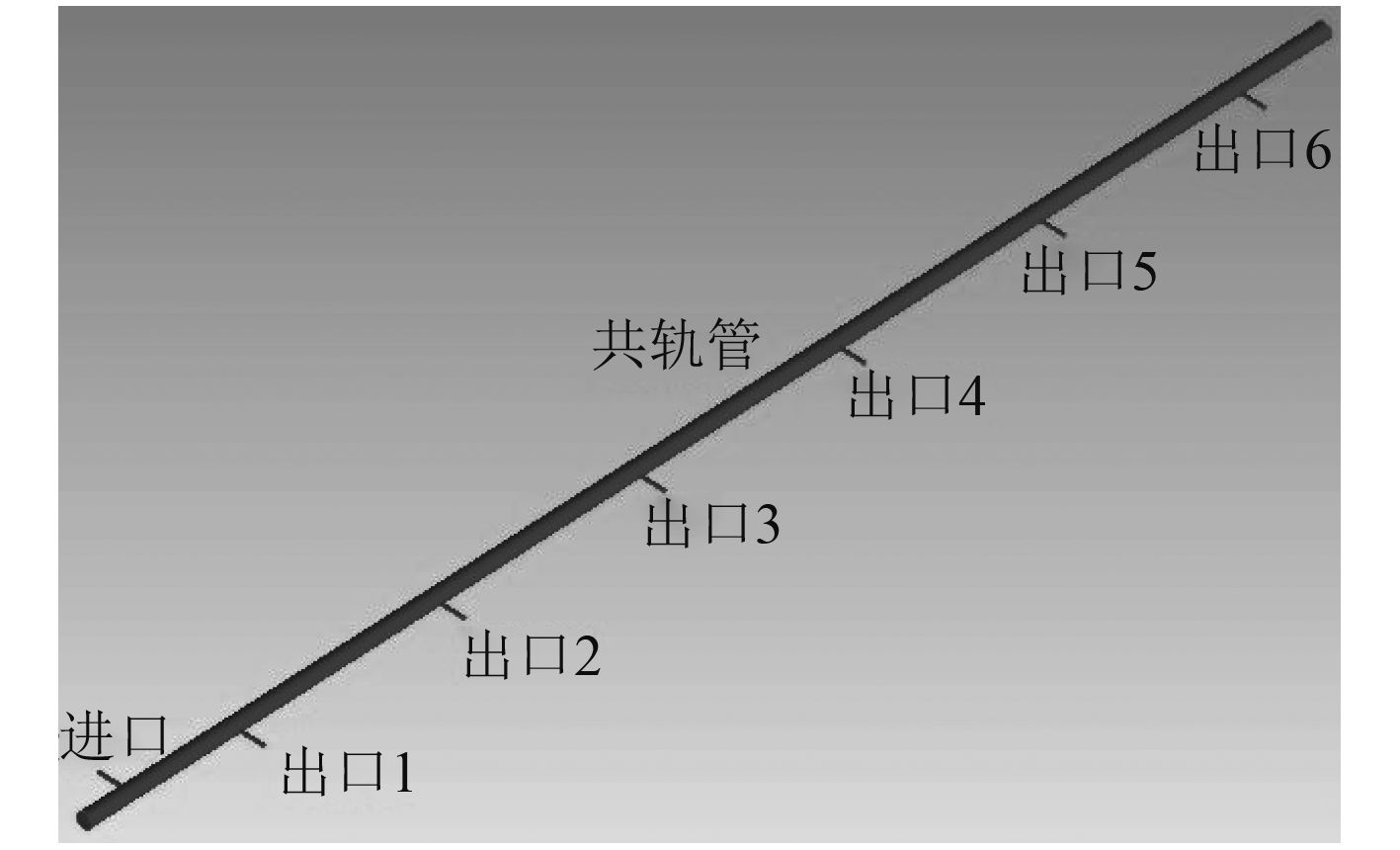图 1 共轨管模型 Fig. 1 The model of common rail
2.2 流动模型网格划分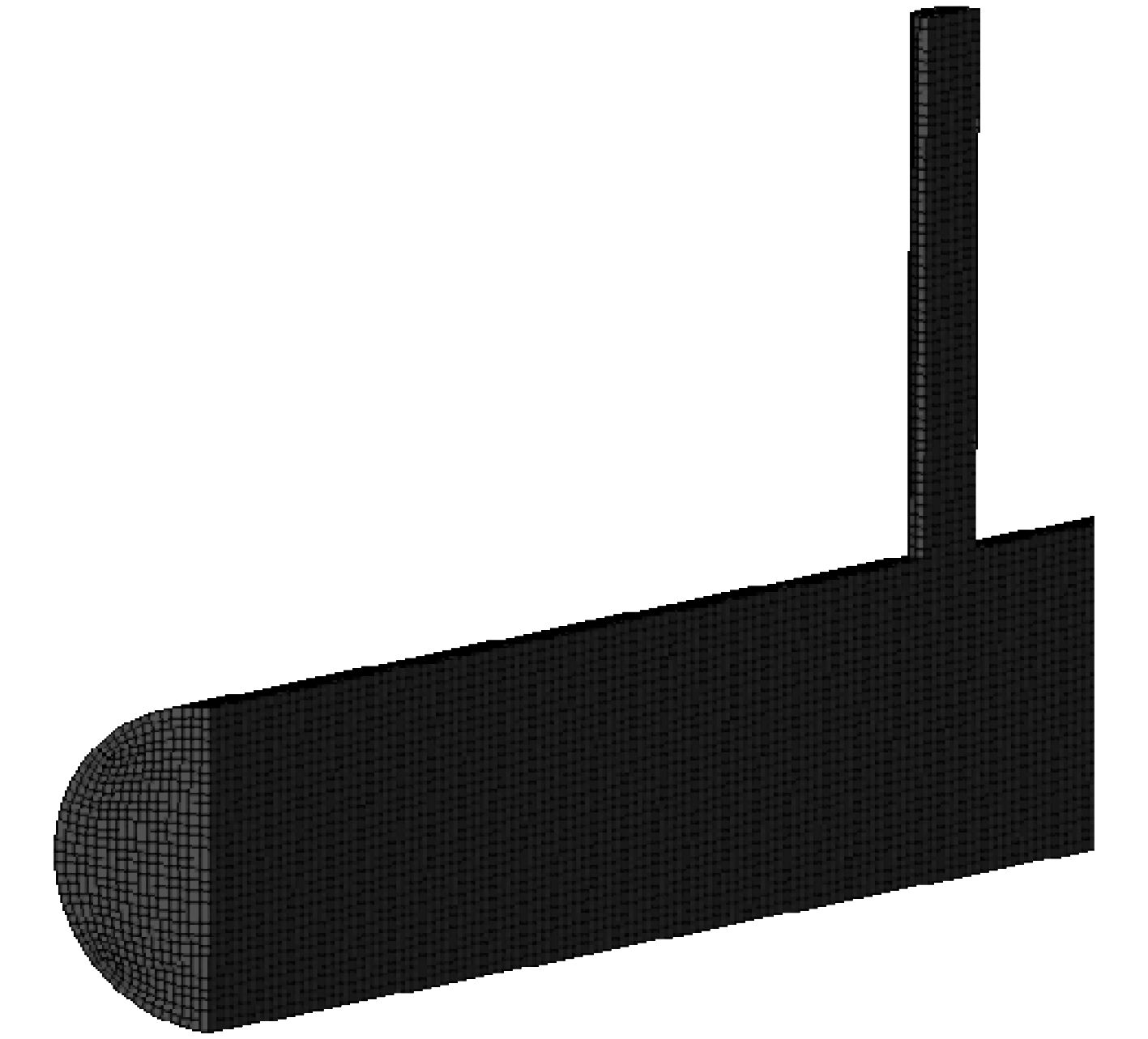图 2 共轨管网格模型 Fig. 2 The mesh model of common rail
2.3 边界条件和初始条件设置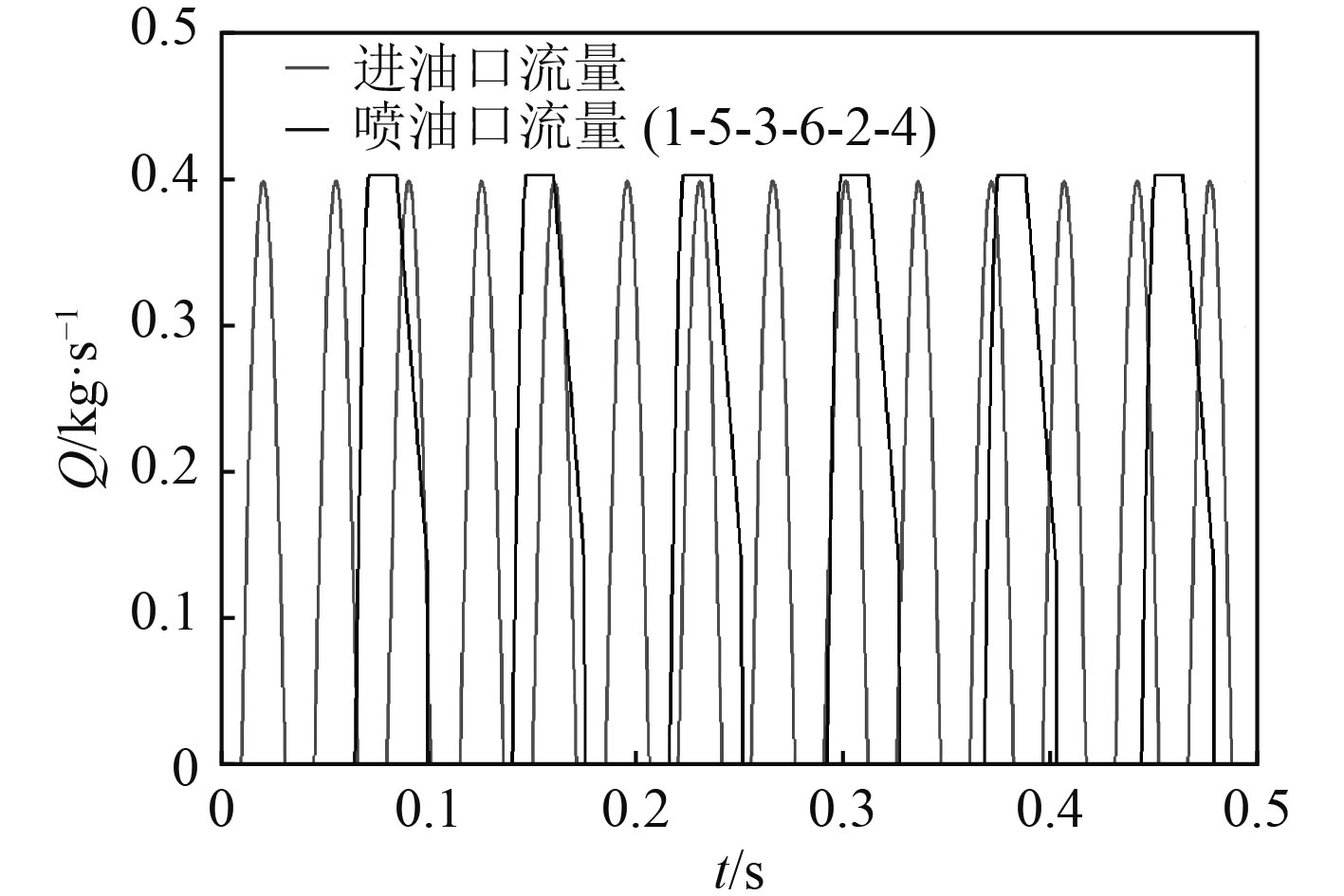图 3 进出口流量曲线 Fig. 3 The flow rate of inlet and outlets
 $Q(t)=0.4\sin (148.188t-148.188{{t}_{0}}){\text{，}}$ (3)
 $$Q(t) = \left\{ \begin{array}{l} 0.405\sin (224.4t - 224.4{t_1}),{t_1} \le t \le {t_2}\\ 0.405,{t_2} \le t \le {t_3}\\ 18(t - {t_4}) - 0.135,{t_3} \le t \le {t_4} \end{array} \right.$$ (4)

2.4 求解器、物理模型、算法的选择

3 结果及分析 3.1 固定流量边界下的轨压波动分析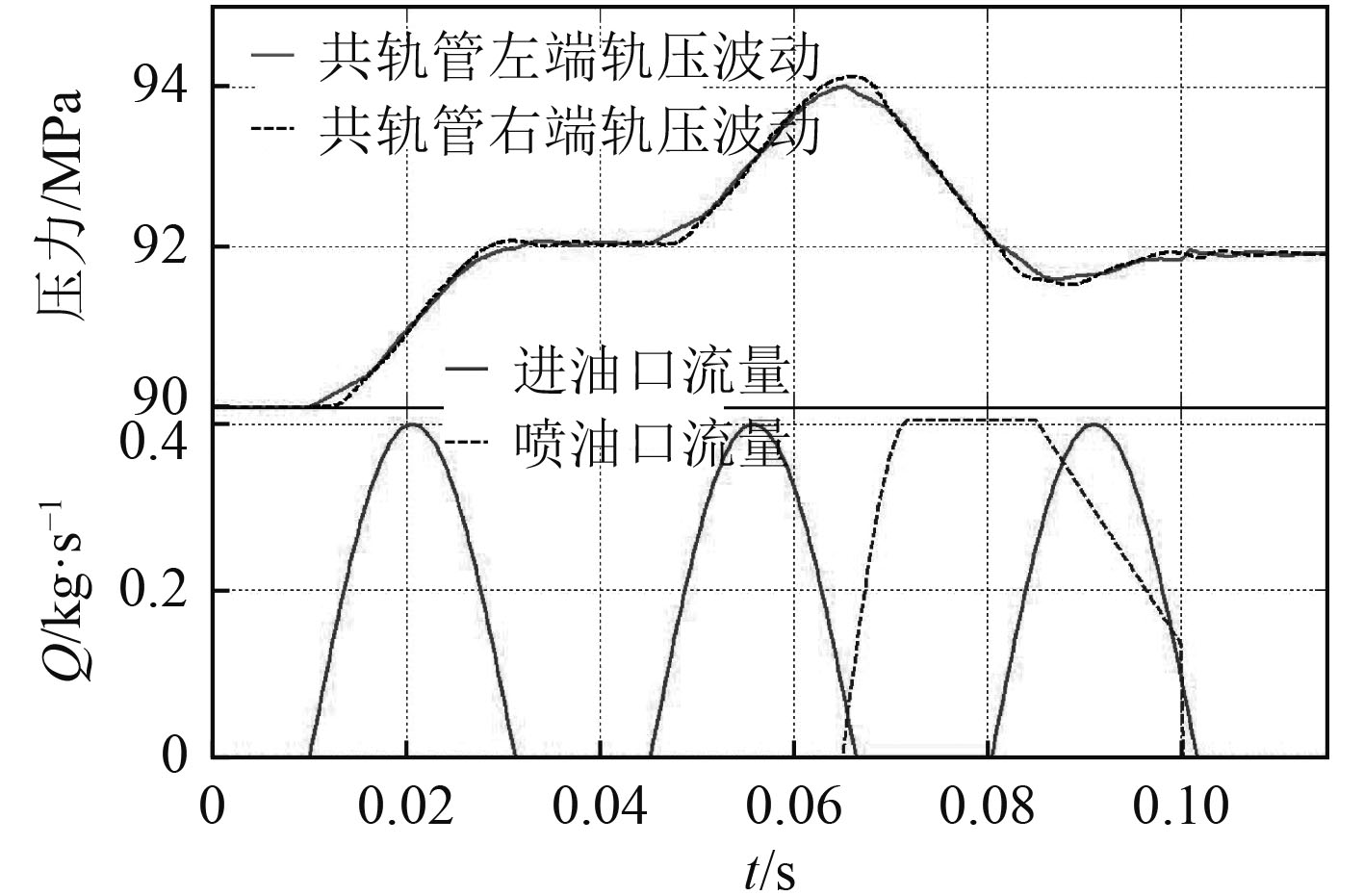图 4 轨压-进出油量对照图 Fig. 4 The comparison between rail pressure and oil quantity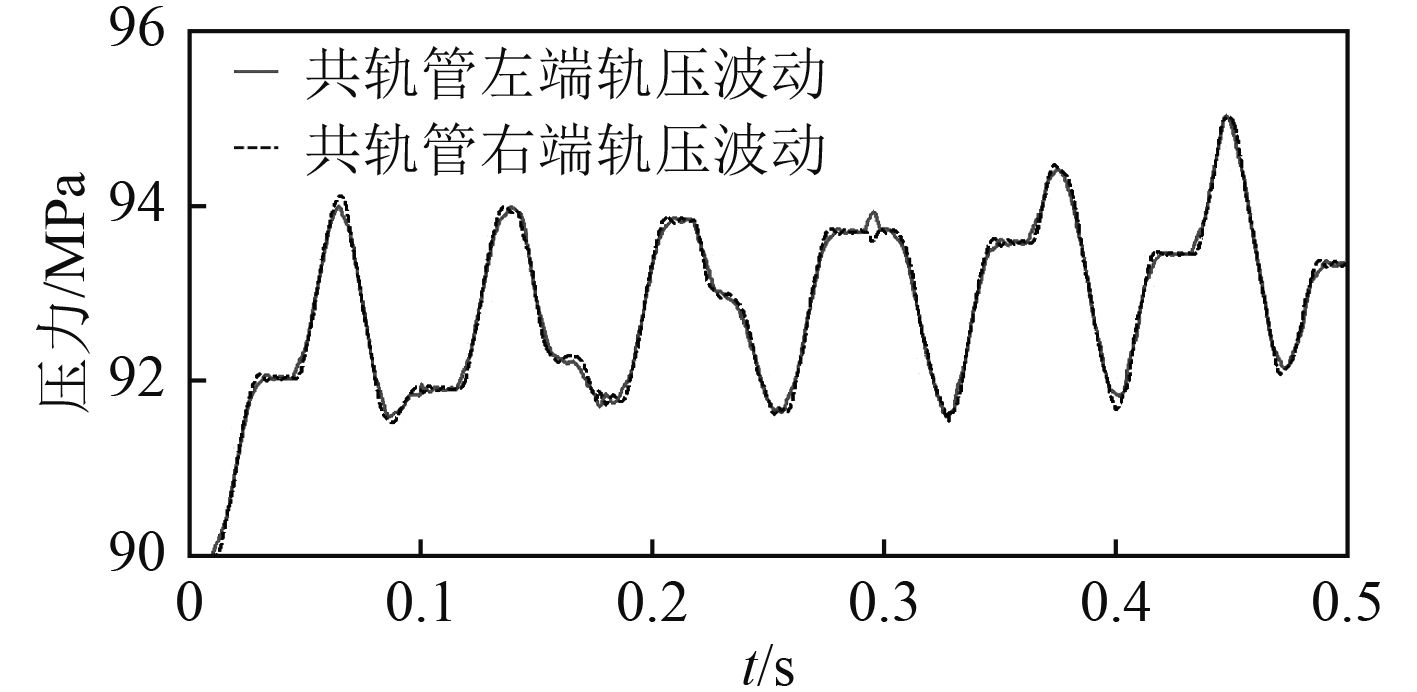图 5 共轨管左右端压力波动 Fig. 5 The fluctuation of rail pressure at the left and right side

3.2 共轨管内燃油流动分析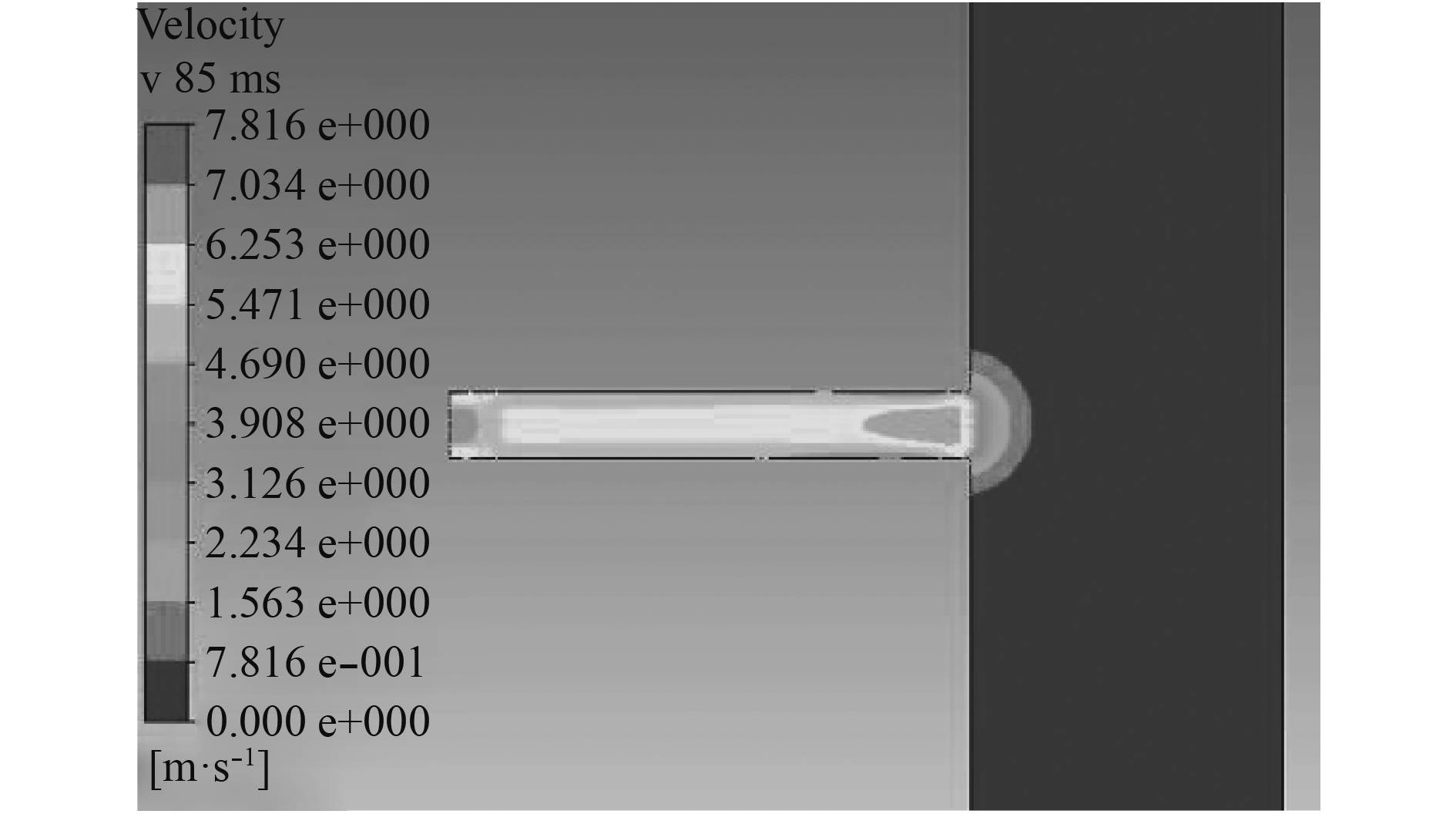图 6 1号出口附近速度分布 Fig. 6 The distribution of velocity near outlet1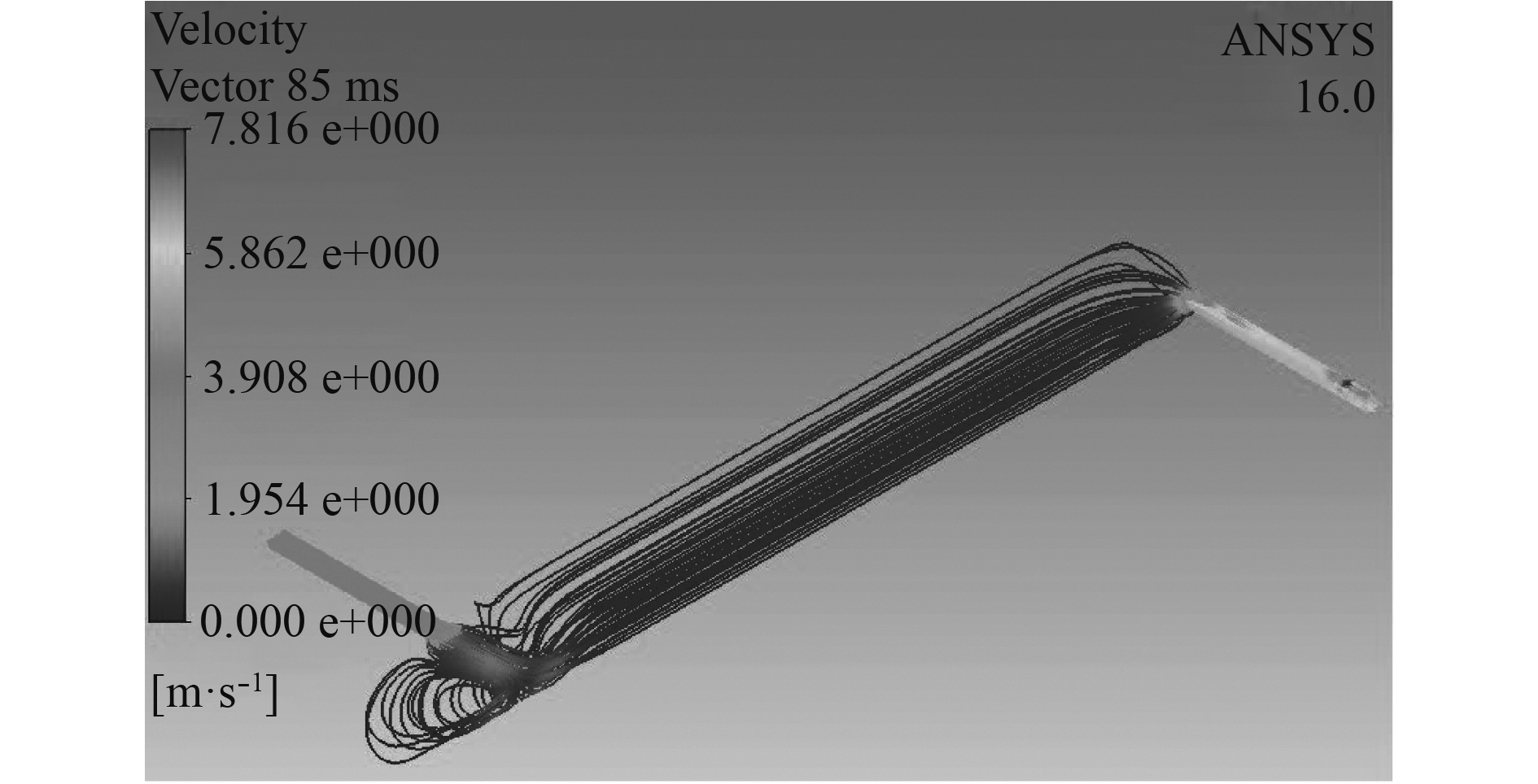图 7 1号出口喷油期间燃油迹线图 Fig. 7 The oil path lines during the injection of outlet1
3.3 轨压波动原因分析

1）柴油机运转时的爆燃现象引起轨压的不稳定；

2）半正弦波供油的二倍频影响；

3）由于节流效应和进出油压差引起的流量变化而产生的反馈影响。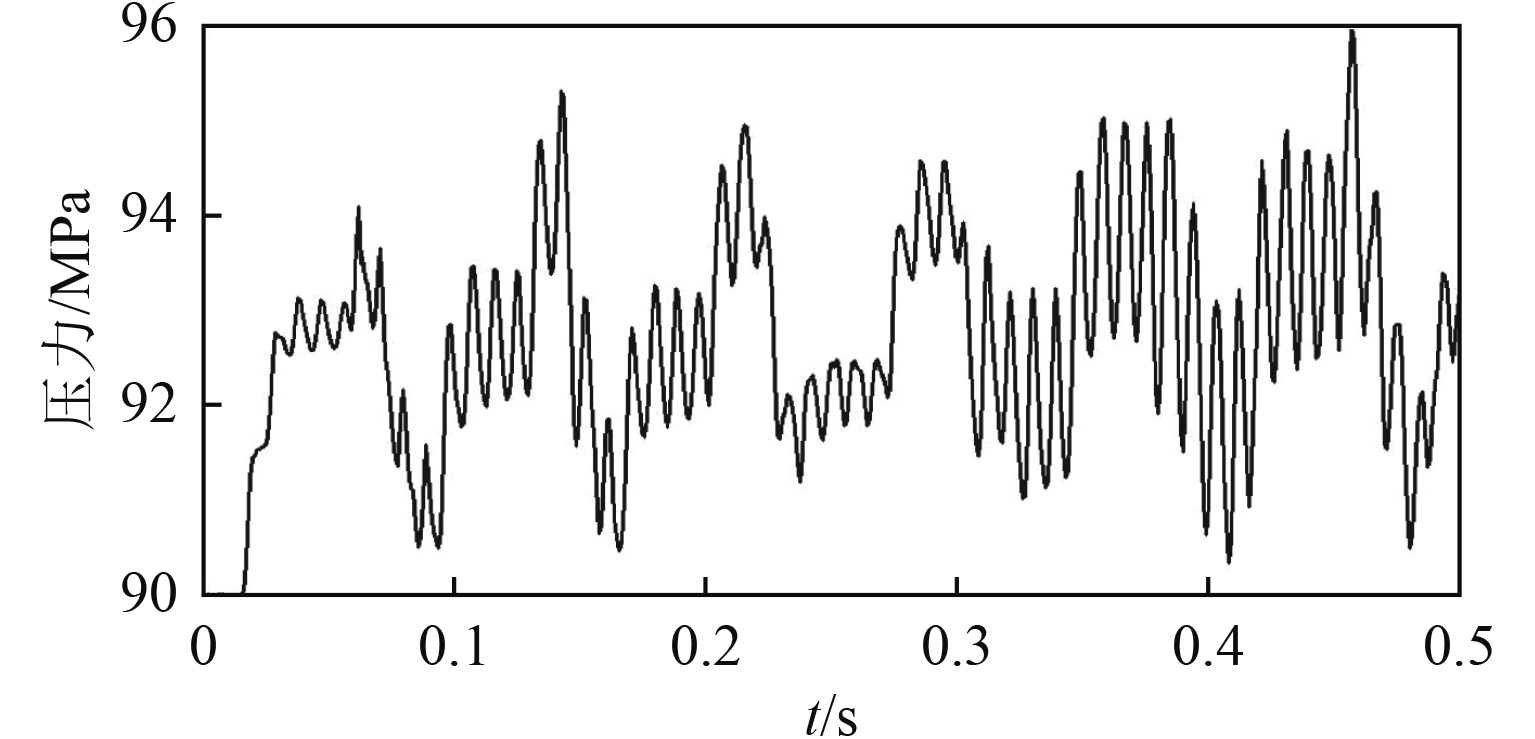图 8 Simulation X模型中的轨压波动 Fig. 8 The rail pressure from model of Simulation X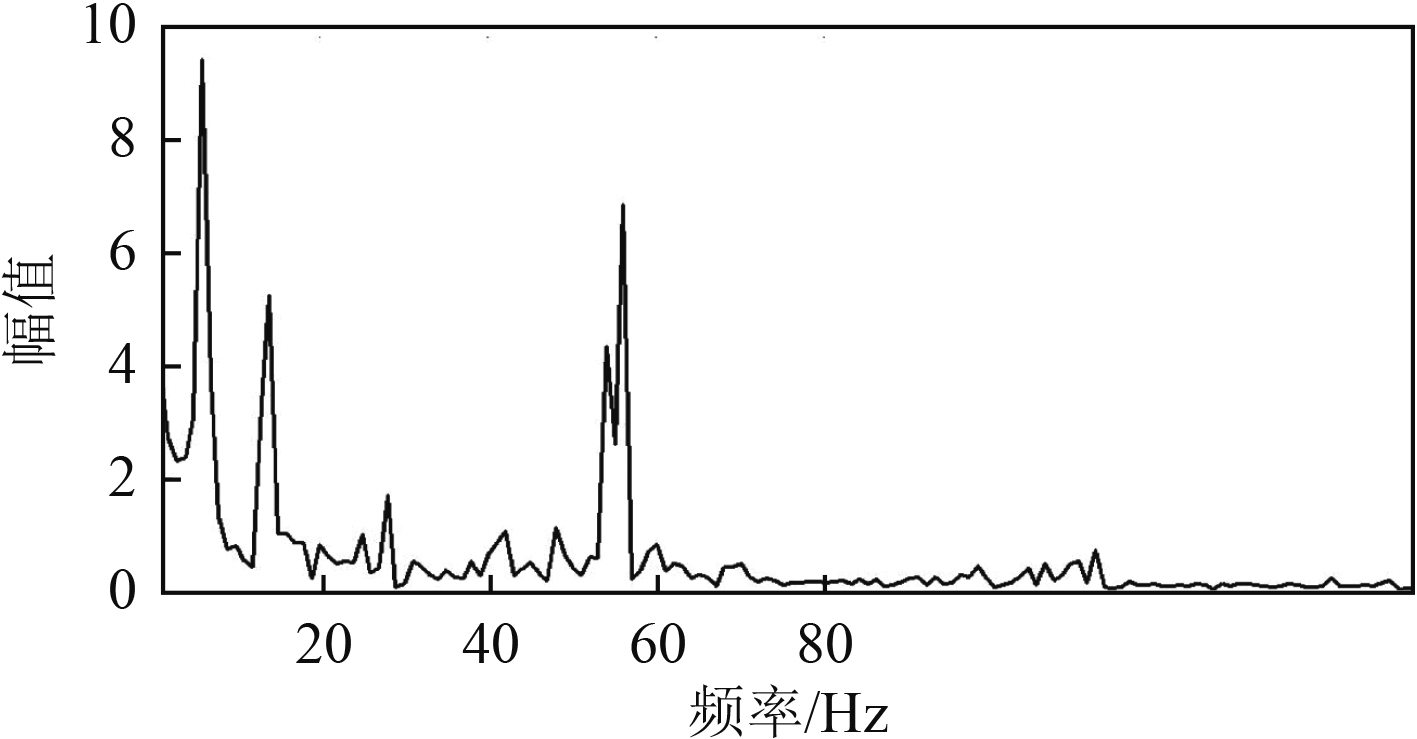图 9 轨压频谱分析图 Fig. 9 The frequency analysis of pressure wave
3.4 节流和压差引起的流量反馈验证

 $Q={{c}_{q}}A\sqrt{\frac{2\left| \Delta p \right|}{\rho }}{\rm{sign}}(\Delta p){\text{。}}$ (5)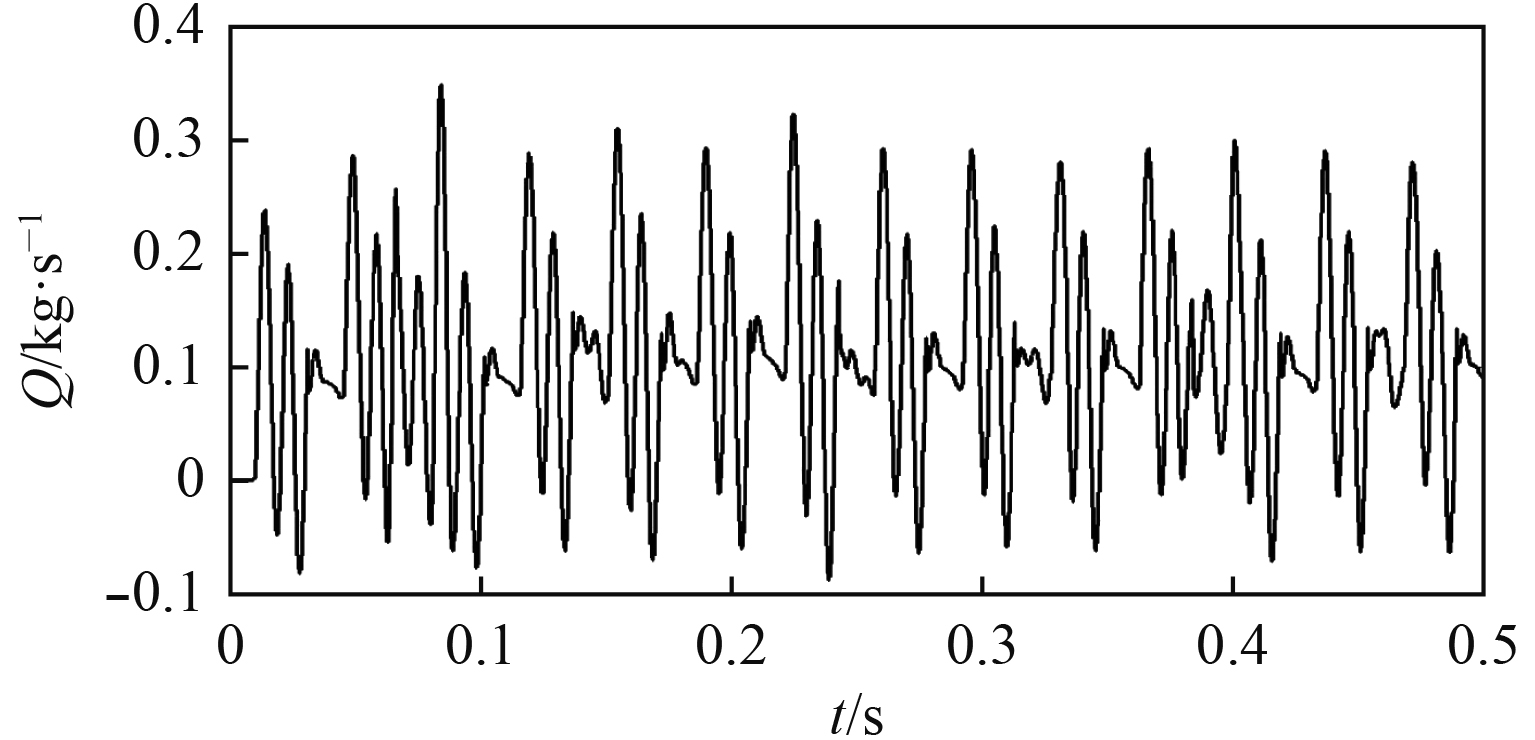图 10 进油口反馈流量 Fig. 10 The feedback flow of the inlet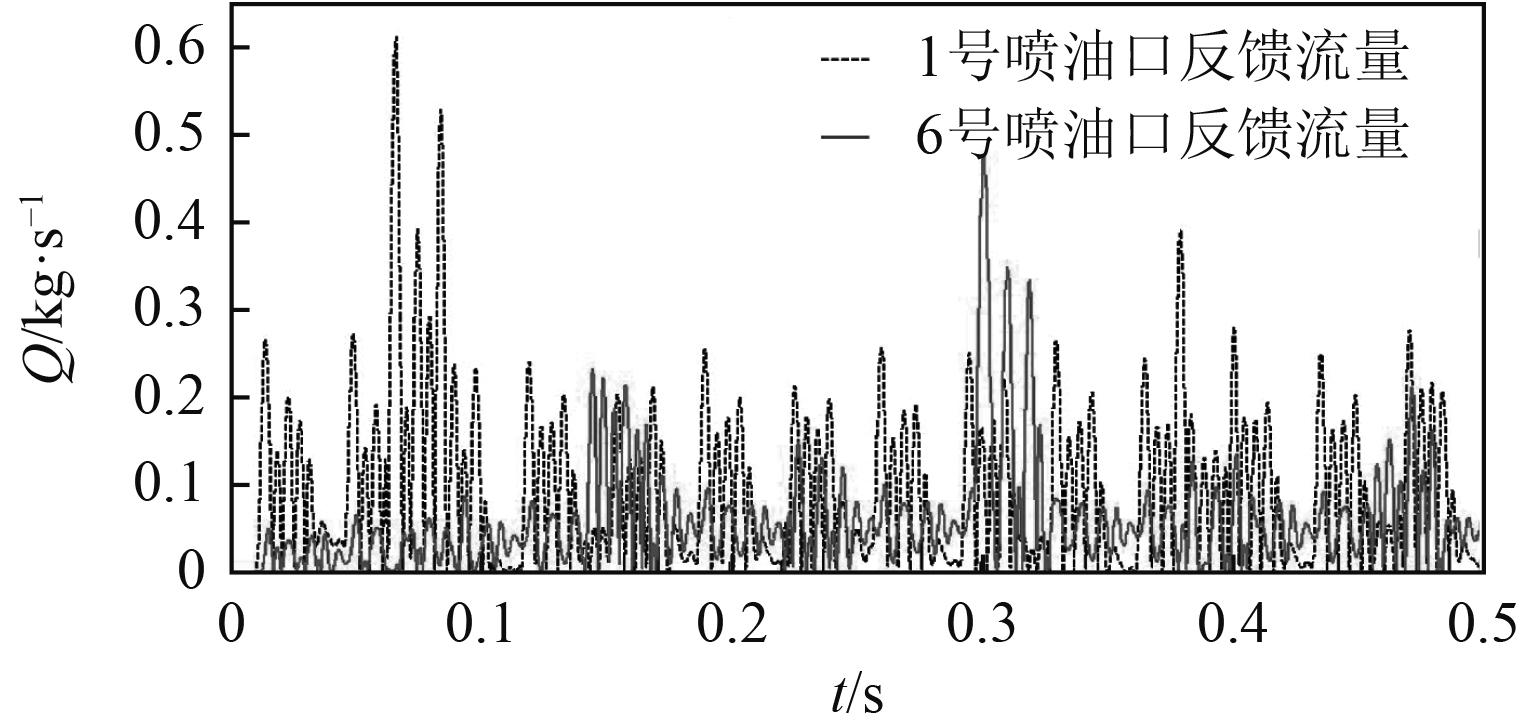图 11 1号和6号喷油口反馈流量 Fig. 11 The feedback flow of outlet 1 and outlet 6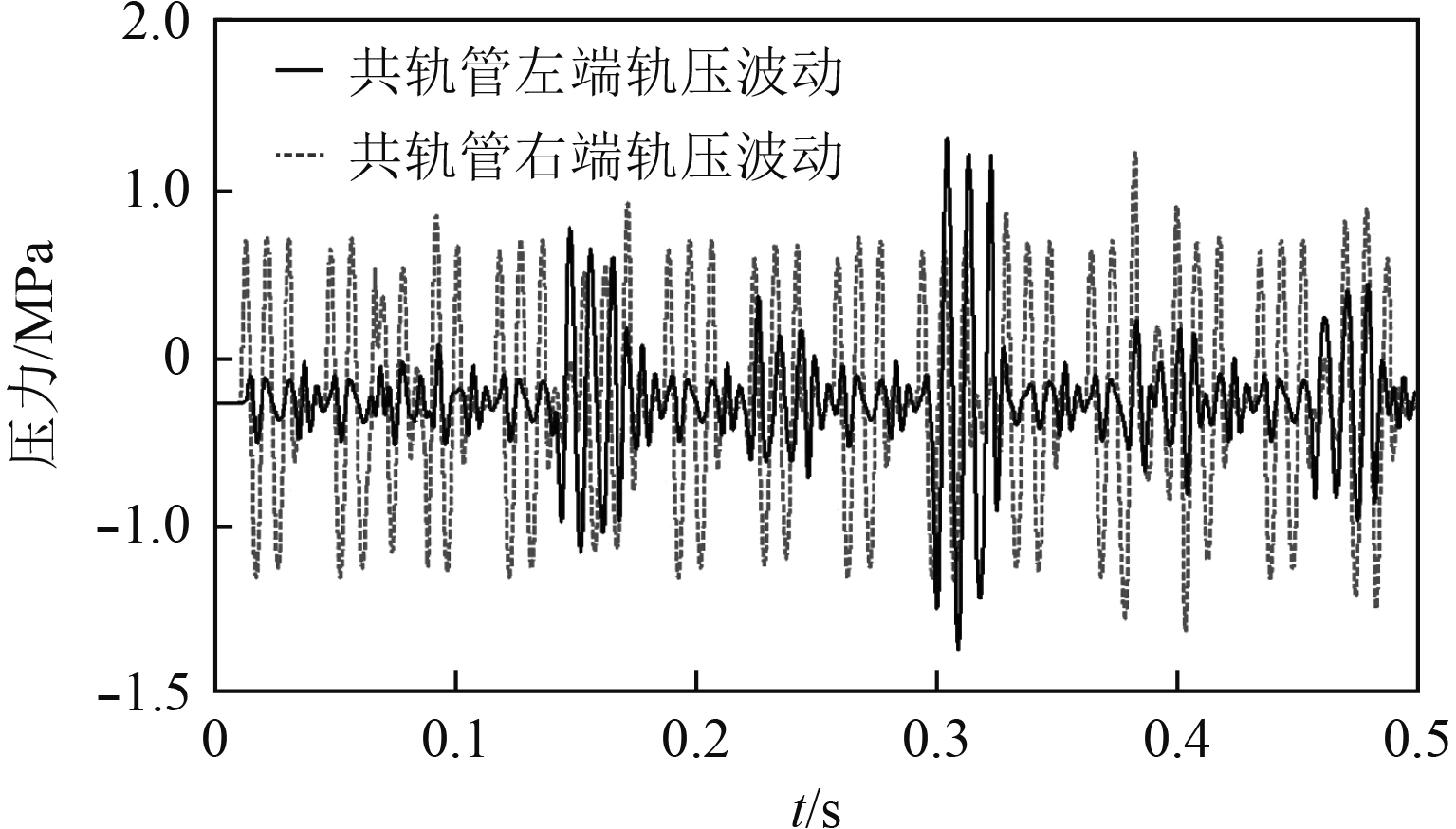图 12 反馈流量引起的轨压波动 Fig. 12 The rail pressure caused by flow feedback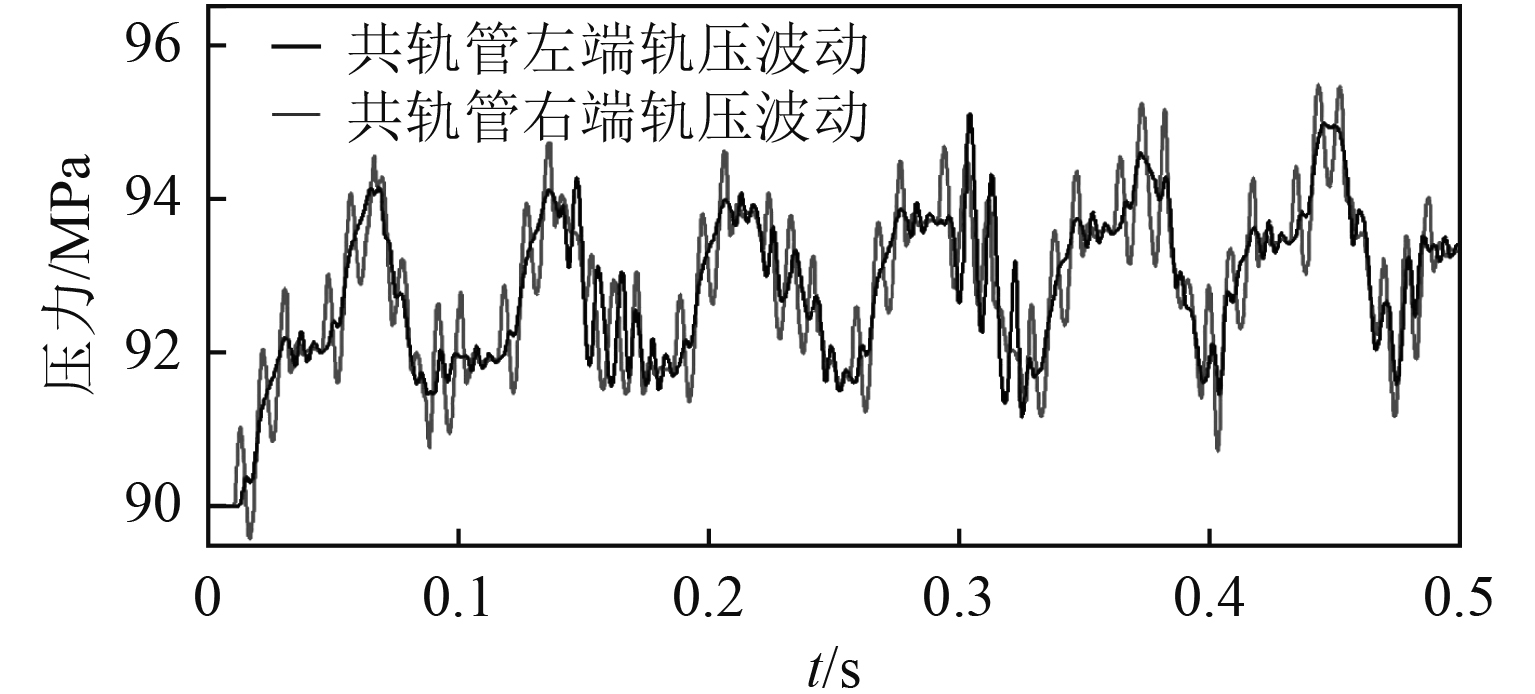图 13 固定流量和反馈流量下的轨压总波动 Fig. 13 The total fluctuation of pressure waves under steady and feedback flow
3.5 共轨压力与喷油量关系分析

 $Q=\alpha T\sqrt{P}{\text{。}}$ (6)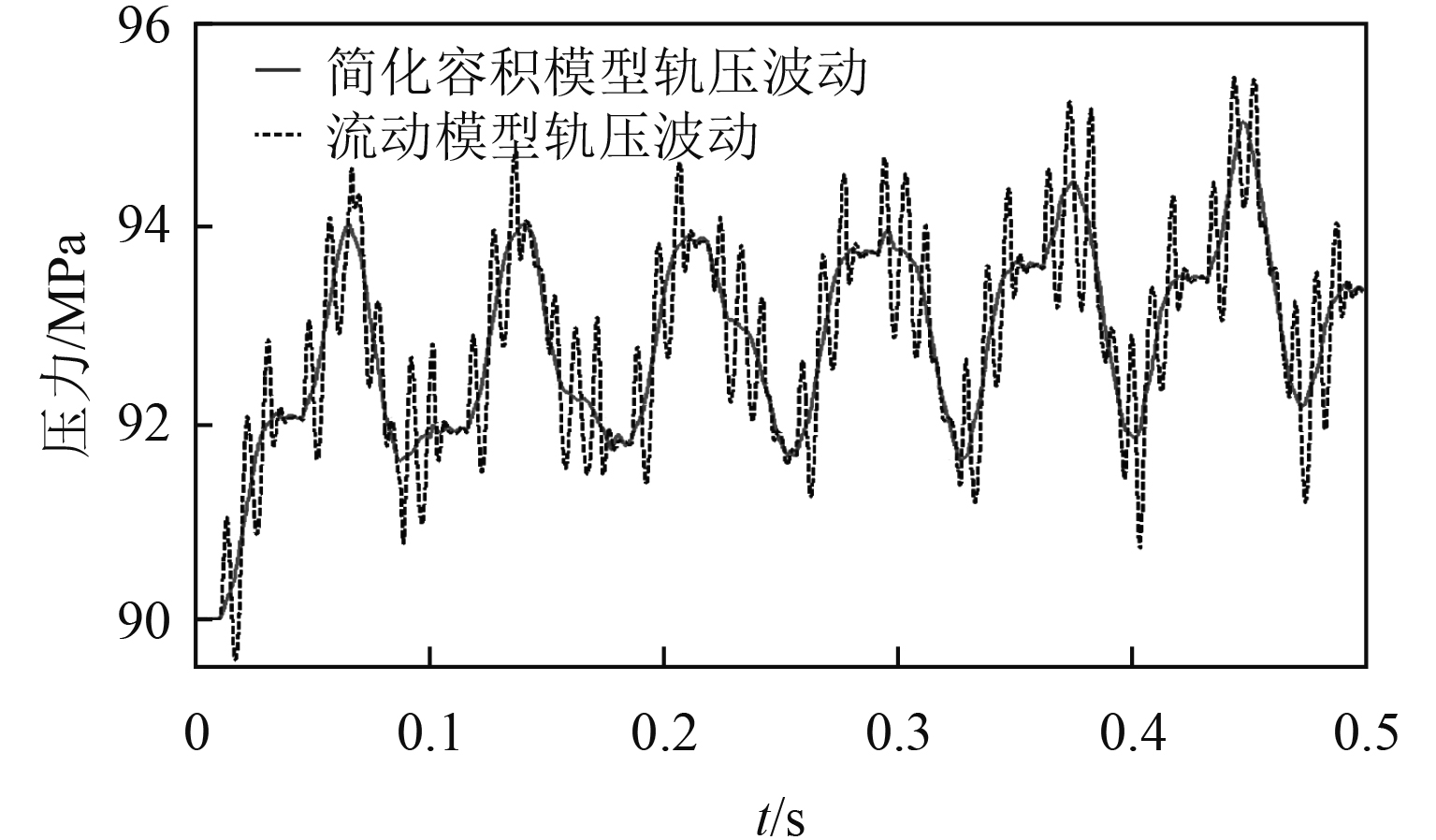图 14 不同模型的轨压波动 Fig. 14 The fluctuation of pressure waves with different models表 1 不同模型下喷油量差异 Tab.1 The difference of injection quantity between different models

4 结　语

1）在运用Fluent等CFD软件对高压燃油在共轨管内的流动进行三维仿真中，将入口和出口的边界条件用物理仿真结果或实际流量数据结合UDF的形式模拟，得到的流动仿真结果将更贴合共轨管内燃油流动实际情况和流场情况，对压力波的模拟也更精准。

2）流动模型通过对共轨管内压力波动模拟和对比，验证了共轨管内存在压力波传递。从高压油泵进油开始，管内压力波从进口端向出口方向传递，左右端压力波动存在明显滞后。共轨管的供油和喷油对压力波动的产生均有作用，轨压波动幅值在2 MPa左右。

3）高压共轨管在供油和喷油时的轨压波动除了供油和喷油是主要影响因素外，由于共轨管节流效应产生的压差会对进出口流量产生反馈影响而使共轨管内压力产生高频波动，高频波动的幅值在1 MPa左右，达到总波动幅值的25%。

4）三维共轨管流动模型结果显示由于压差反馈流量的存在，在共轨管内不同位置的压力差值最大可达整体压力波动的75%，在不同喷油脉宽内所引起的喷油量差值最大可达到20%，因此共轨压力的这一高频波动不可忽视。三维流动模型不仅能够检测共轨管内流场情况，其结果对轨压的精准控制也有更加具体的意义。

  平涛, 徐建新, 方文超, 等. 船用柴油机高压共轨系统动态特性研究[J]. 船舶工程, 2009, 31(3): 7-10. PING Tao, XU Jian-xin, FANG wen-chao, et al. Experimental investigation of high pressure common rail system for marine diesel engine[J]. Ship Engineering, 2009, 31(3): 7-10. DOI:10.3969/j.issn.1000-6982.2009.03.003  王书义. 不可压缩流体三维流动的数值计算[J]. 兵工学报坦克装甲车与发动机分册, 1992(2): 5-10. WANG Shu-yi. Numerical calculation of three-dimensional flow of incompressible fluid[J]. Acta Armamentarii, 1992(2): 5-10.  王海燕, 张伟, 周海涛. 船用大型低速电控柴油机的容积法模型[J]. 内燃机学报, 2008, 26(5): 452-456. WANG Hai-yan, ZHANG Wei, ZHOU Hai-tao. Volumetric model of a large-scale low-speed electronically controlled marine diesel engine[J]. Transactions of CSICE, 2008, 26(5): 452-456. DOI:10.3321/j.issn:1000-0909.2008.05.011  王永坚, 李斯钦, 王海燕. 大型低速船用智能柴油机燃油共轨喷射系统的建模与动态仿真[J]. 船舶工程, 2012, 34(4): 45-48. WANG Yong-jian, LI Si-qing, WANG Hai-yan. Modeling and dynamic simulation for fuel common rail injection system of large low-speed marine intelligent diesel engine[J]. Ship Engineering, 2012, 34(4): 45-48.  王永坚, 杨国豪, 李斯钦. 船用电控柴油机燃油共轨系统与轨压力仿真[J]. 中国航海, 2013, 36(2): 22-27. WANG Yong-jian, YANG Guo-hao, LI Si-qin. Simulation for fuel common rail systems and rail pressure about electronically controlled marine diesel engines[J]. Navigation of China, 2013, 36(2): 22-27. DOI:10.3969/j.issn.1000-4653.2013.02.006  屠星星, 王俊雄, 黄丹青, 等. 基于SimulationX的船用高压共轨燃油系统仿真 [J]. 舰船科学技术, 2015(3): 81-84. TU Xing-xing, WANG Jun-xiong, HUANG Dan-qing, et al. Simulation of the marine high-pressure common rail fuel system based on simulationX [J]. Ship Science and Technology, 2015(3): 81-84. DOI:10.3404/j.issn.1672-7649.2015.03.017  王书义. 用SIMPLE算法计算高压油管内的燃油流动[J]. 兵工学报坦克装甲车与发动机分册, 1989(2): 2-9. WANG Shu-yi. Calculation of fuel flow in high pressure fuel pipe by means of simple method[J]. Acta Armamentarii, 1989(2): 2-9.  陆方迪. 高压共轨系统轨内压力波特性的仿真研究[D]. 北京: 北京交通大学, 2012. LU Fang-di. Simulation of characteristics of pressure fluctuation in the common rail of CRS[D]. Beijing: Beijing Jiao Tong University, 2012.